Updating search results...

# 29 Results

View
Selected filters:
• NC.Math.8.G.5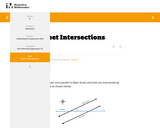Unrestricted Use
CC BY
Rating
0.0 stars

This is a task from the Illustrative Mathematics website that is one part of a complete illustration of the standard to which it is aligned. Each task has at least one solution and some commentary that addresses important aspects of the task and its potential use.

Subject:
Mathematics
Material Type:
Activity/Lab
Provider:
Illustrative Mathematics
Provider Set:
Illustrative Mathematics
Author:
Illustrative Mathematics
05/03/2019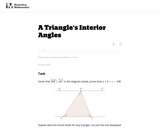Unrestricted Use
CC BY
Rating
0.0 stars

This is a task from the Illustrative Mathematics website that is one part of a complete illustration of the standard to which it is aligned. Each task has at least one solution and some commentary that addresses important asects of the task and its potential use. Here are the first few lines of the commentary for this task: Given that $\overleftrightarrow{DE}\parallel\overleftrightarrow{AC}$ in the diagram below, prove that $a + b + c = 180.$ Explain why this result holds ...

Subject:
Mathematics
Material Type:
Activity/Lab
Provider:
Illustrative Mathematics
Provider Set:
Illustrative Mathematics
Author:
Illustrative Mathematics
05/15/2013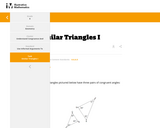Unrestricted Use
CC BY
Rating
0.0 stars

This is a task from the Illustrative Mathematics website that is one part of a complete illustration of the standard to which it is aligned. Each task has at least one solution and some commentary that addresses important aspects of the task and its potential use.

Subject:
Mathematics
Material Type:
Activity/Lab
Provider:
Illustrative Mathematics
Provider Set:
Illustrative Mathematics
Author:
Illustrative Mathematics
05/03/2019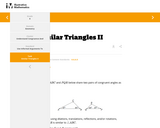Unrestricted Use
CC BY
Rating
0.0 stars

This is a task from the Illustrative Mathematics website that is one part of a complete illustration of the standard to which it is aligned. Each task has at least one solution and some commentary that addresses important aspects of the task and its potential use.

Subject:
Mathematics
Material Type:
Activity/Lab
Provider:
Illustrative Mathematics
Provider Set:
Illustrative Mathematics
Author:
Illustrative Mathematics
05/03/2019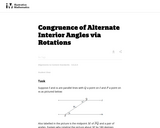Unrestricted Use
CC BY
Rating
0.0 stars

This is a task from the Illustrative Mathematics website that is one part of a complete illustration of the standard to which it is aligned. Each task has at least one solution and some commentary that addresses important asects of the task and its potential use. Here are the first few lines of the commentary for this task: Suppose $\ell$ and $m$ are parallel lines with $Q$ a point on $\ell$ and $P$ a point on $m$ as pictured below: Also labelled in the picture is the midp...

Subject:
Mathematics
Material Type:
Activity/Lab
Provider:
Illustrative Mathematics
Provider Set:
Illustrative Mathematics
Author:
Illustrative Mathematics
10/14/2013Conditional Remix & Share Permitted
CC BY-NC-SA
Rating
0.0 stars

This 8th grade Math&nbsp;parent guide&nbsp;explains the content in straightforward terms so they can support their children&rsquo;s learning at home and will&nbsp;encourage&nbsp;caretaker engagement&nbsp;with lessons.

Subject:
Mathematics
Material Type:
Reference Material
Author:
Kelly Rawlston
Letoria Lewis
01/10/2022Conditional Remix & Share Permitted
CC BY-NC-SA
Rating
0.0 stars

Our Teacher Guides are meant to support the use of our online course and unit content. Please use these to accompany the use of our content and for ideas to support struggling learners, those needing extension and for additional resources.

Subject:
Mathematics
Material Type:
Curriculum
Teaching/Learning Strategy
Author:
Kelly Rawlston
Letoria Lewis
05/16/2022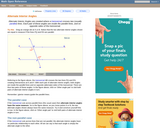Rating
0.0 stars

An interactive applet and associated web page that demonstrate the alternate interior angles that are formed where a transversal crosses two lines. The applets shows the two possible pairs of angles alternating when in animation mode. By dragging the three lines, it can be seen that the angles are congruent only when the lines are parallel. When not in animated mode, there is a button that alternates the two pairs of angles. The text on the page discusses the properties of the angle pairs both in the parallel and non-parallel cases. Applet can be enlarged to full screen size for use with a classroom projector. This resource is a component of the Math Open Reference Interactive Geometry textbook project at http://www.mathopenref.com.

Subject:
Geometry
Mathematics
Material Type:
Simulation
Provider:
Math Open Reference
Author:
John Page
05/07/2019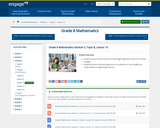Conditional Remix & Share Permitted
CC BY-NC-SA
Rating
0.0 stars

In this lesson, students know an informal proof of the Angle-Angle (AA) criterion for similar triangles. Students present informal arguments as to whether or not triangles are similar based on Angle-Angle criterion.

Subject:
Mathematics
Material Type:
Lesson Plan
Provider:
EngageNY
02/16/2017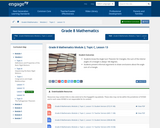Conditional Remix & Share Permitted
CC BY-NC-SA
Rating
0.0 stars

In this lesson, students know the Angle Sum Theorem for triangles; the sum of the interior angles of a triangle is always 180 degrees. Students present informal arguments to draw conclusions about the angle sum of a triangle.

Subject:
Mathematics
Material Type:
Lesson Plan
Provider:
EngageNY
02/16/2017Unrestricted Use
CC BY
Rating
0.0 stars
Rating
0.0 stars
Subject:
Mathematics
Material Type:
Lesson Plan
Author:
Danielle Flores
11/06/2019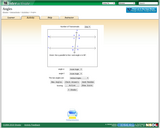Rating
0.0 stars

In this interactive, students demonstrate their understanding of angle vocabulary.

Subject:
Mathematics
Material Type:
Interactive
Provider:
Shodor Education Foundation
03/24/2017Rating
0.0 stars

The students will be split into small, cooperative groups and build a city following specific guidelines. The students will learn how a city is constructed with angles, intersections, parallel, intersecting, and transversal streets. They will understand that buildings are located at particular points which are considered alternate interior or exterior angles, corresponding angles, etc.

Subject:
Mathematics
Material Type:
Lesson Plan
Provider:
Alabama Learning Exchange
Author:
Sellers Kennington
02/26/2019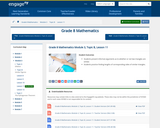Conditional Remix & Share Permitted
CC BY-NC-SA
Rating
0.0 stars

In this lesson, students present informal arguments as to whether or not two triangles are similar. Students practice finding lengths of corresponding sides of similar triangles.

Subject:
Mathematics
Material Type:
Lesson Plan
Provider:
EngageNY
02/16/2017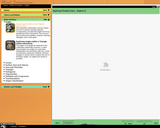Rating
0.0 stars

For this interactive, students explore vertically opposite, corresponding, and alternate angles formed by parallel lines and a transversal. The resource also includes print activities, solutions, learning strategies, and a math game.

Subject:
Mathematics
Material Type:
Interactive
Provider:
Math Interactives
03/24/2017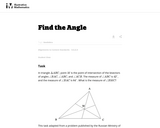Unrestricted Use
CC BY
Rating
0.0 stars

This task requires students to find the measure of an angle.

Subject:
Mathematics
Material Type:
Activity/Lab
Provider:
Illustrative Mathematics
Provider Set:
Illustrative Mathematics
Author:
Illustrative Mathematics
05/01/2012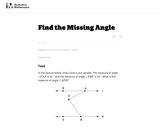Unrestricted Use
CC BY
Rating
0.0 stars

This task "Uses facts about supplementary, complementary, vertical, and adjacent angles in a multi-step problem to write and solve simple equations for an unknown angle in a figure (7.G.5)" except that it requires students to know, in addition, something about parallel lines, which students will not see until 8th grade.

Subject:
Mathematics
Material Type:
Activity/Lab
Provider:
Illustrative Mathematics
Provider Set:
Illustrative Mathematics
Author:
Illustrative Mathematics
05/01/2012Rating
0.0 stars

This is a set of four, one-page problems about the distance craft travel on Mars. Learners will use the Pythagorean Theorem to determine distance between a series of hypothetical exploration sites within Gale Crater on Mars. Options are presented so that students may learn about the Mars Science Laboratory (MSL) mission through a NASA press release or by viewing a NASA eClips video [6 min.]. This activity is part of the Space Math multi-media modules that integrate NASA press releases, NASA archival video, and mathematics problems targeted at specific math standards commonly encountered in middle school.

Subject:
Science
Material Type:
Lesson
Provider:
NASA
Provider Set:
Space Math
07/31/2019Rating
0.0 stars

Students will learn about translations, reflections, and rotations in the plane and, more importantly, how to use them to precisely define the concept of congruence.

Subject:
Mathematics
Material Type:
Unit of Study
Provider:
EngageNY
Author:
EngageNY
02/26/2019Rating
0.0 stars

Students will learn about dilation and similarity and apply that knowledge to a proof of the Pythagorean Theorem based on the Angle-Angle criterion for similar triangles.

Subject:
Mathematics
Material Type:
Unit of Study
Provider:
EngageNY
Author:
EngageNY